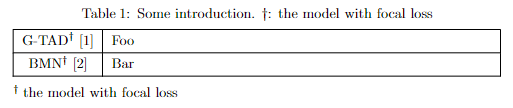Which package to use? Below is some package you must know:

• tabular: The basic one [not recommended].
• array: A extension of tabular. Depended by most of the other table packages.
• tabularx: Introduce the useful X column. Recommended.
• booktabs: Professional looking. Specifically designed for tables without vertical lines.
• tabularray: A new LaTeX3 package using a new writting logic. Well maintained and powerful. Recommended. You may read my post about it.

## Miscellaneous

### X column in the tabularx

One important feature of the tabularx is the column type X, which automatically expands in order to make the table as wide as specified, e.g., \textwidth. Below are the examples:

\begin{tabularx}{0.5\textwidth}{|l|l|l|}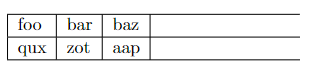\begin{tabularx}{0.5\textwidth}{|X|X|X|}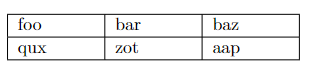However, it’s quite tricky to do alignment in X columns:

{>{\raggedright\arraybackslash}X} % align left
{>{\centering\arraybackslash}X}   % align center
{>{\raggedleft\arraybackslash}X}  % align right


Note that the X column and the c l r columns can be used in the same table:

\begin{tabularx}{\textwidth}{l l l l l *{5}{>{\centering\arraybackslash}X}}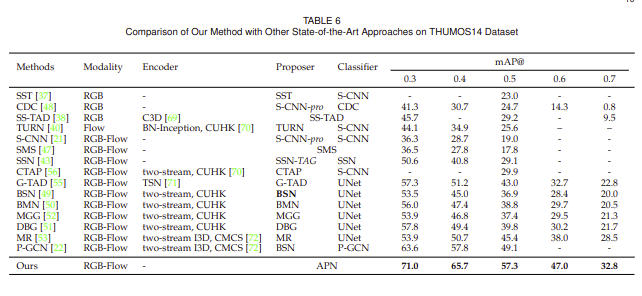In the above example, only the width of the rightest five columns, i.e. the X columns, are auto-ajusted.

Altough the X column is good, there is a drawback that all X columns in the same table share the same width. I think that ideal X columns should have width proportional to their text contained. Below is an example:

\begin{tabularx}{\textwidth}{|X| X| X| X| X| X |X| X| X| X| X|}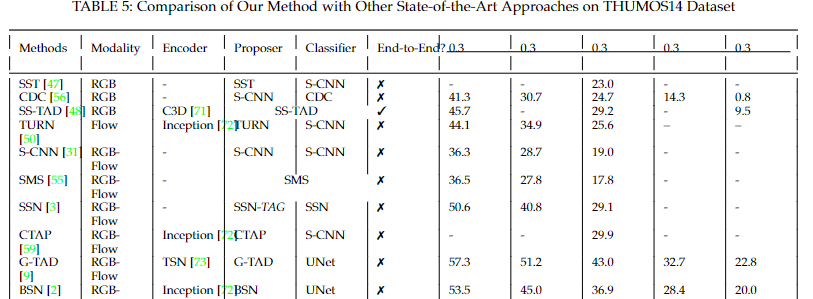As we can see that all X column share the same width. A better width arrangement should be that the columns with long texts have larger width. There are two ways to do that referring to this answer:

• Using \extracolsep{\fill} inside tabular*.
• The X[-1] column in the tabularray package.

### Bold \hline

\usepackage{makecell}

% inside table
\Xhline{1.5pt}
\Xhline{2\arrayrulewidth}


### Multiple rows and columns

%multi-column
\multicolumn{number cols}{align}{text} % align: l,c,r

%multi-row
\usepackage{multirow}
\multirow{number rows}{width}{text}
% Using * as width, the text argument’s natural width is used.


### Repeated columns

*{num_repeated}{alignment} % see the above example


### Unbreackable text \mbox

Before: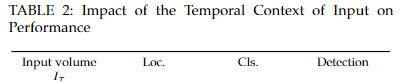After:

\mbox{Input volume $I_{\tau}$} & Loc. &  Cls. & Detection\\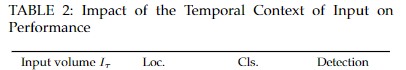### Custom alignment on specific cell

\multicolumn{1}{|r|}{Item3}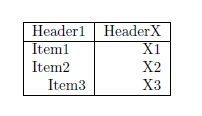## Superscript in table

Either using Test$^\dag$ and expalining the superscript in the Table title, or using the \TblrNote{$\dag$} in the tabularray package (longtblr) and describing the superscripts in notes note{$\dag$} = {text} under the table.

\renewcommand\TblrOverlap{#1} % change the overlap style, you may comment this line to check the difference.
\begin{longtblr}[
caption = {Some introduction. $\dag$: the model with focal loss},
note{$\dag$} = {the model with focal loss},
]{
colspec = {c X},
hlines,
vlines,
}
G-TAD$^\dag$  & Foo \\
BMN\TblrNote{$\dag$}  & Bar\\
\end{longtblr}